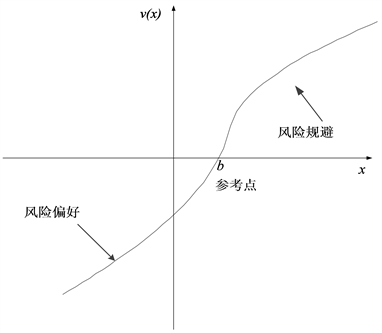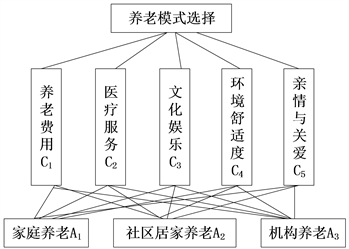﻿ 基于犹豫模糊集的养老模式选择决策研究

# 基于犹豫模糊集的养老模式选择决策研究Research on Decision-Making Decision of Pension Model Based on Hesitant Fuzzy Set

Abstract: To effectively alleviate the burden of pensions brought about by the aging of the population, this paper provides an hesitant fuzzy multi-criteria decision analysis method based on the improved prospect theory, and applies it to the decision of the choice of pension mode. First, we construct an evaluation index system for the old-age model and establish a hesitant fuzzy evaluation matrix. Secondly, based on the irrational behavior of decision makers, the improved prospect theory is introduced to construct an improved foreground decision matrix. For the problem of definite power, an optimization model that comprehensively considers the main and objective factors is established. Then, combined with the improved foreground decision matrix and criterion weights, a hesitant fuzzy multi-criteria decision making method based on improved foreground theory is proposed. Based on this method, the decision-making problem of the pension model is studied, and an effective analysis method is provided for such problems, and the optimization decision of such problems is made through examples.

1. 引言

2. 犹豫模糊集及改进的前景理论

2.1. 犹豫模糊集

${h}_{A}\left(X\right)=\left\{〈x,{h}_{A}\left(x\right)〉|x\in X\right\}$

$s\left(h\left(x\right)\right)=\frac{1}{{l}_{h}}{\sum }_{\gamma \in h\left(x\right)}\gamma$ (1)

2.2. 改进前景理论

2.2.1. 前景理论

1979年，Kahneman和Tversky  提出前景理论(Prospect Theory)，该理论由价值函数和概率权重函数共同组成，即：

$V=\underset{i=1}{\overset{n}{\sum }}w\left({p}_{i}\right)v\left(\Delta {x}_{i}\right)$ (2)

$w\left(p\right)=\left\{\begin{array}{l}\frac{{p}^{\chi }}{{\left({p}^{\chi }+{\left(1-p\right)}^{\chi }\right)}^{1/\chi }},\text{ }\text{ }\text{ }\text{ }\text{ }\text{ }\text{\hspace{0.17em}}\Delta x\ge 0\\ \frac{{p}^{\delta }}{{\left({p}^{\delta }+{\left(1-p\right)}^{\delta }\right)}^{1/\delta }},\text{ }\text{ }\text{ }\text{ }\text{ }\text{ }\text{\hspace{0.17em}}\Delta x<0\end{array}$ (3)

$v\left(\Delta x\right)=\left\{\begin{array}{l}{\left(\Delta x\right)}^{\alpha },\text{ }\text{ }\text{ }\text{ }\text{ }\text{ }\text{ }\text{ }\text{ }\Delta x\ge 0\\ -\theta {\left(-\Delta x\right)}^{\beta },\text{ }\text{ }\text{ }\text{ }\text{ }\text{\hspace{0.17em}}\Delta x<0\end{array}$ (4)Figure 1. Outlook value function

2.2.2. 改进前景理论

$v\left(\Delta x\right)=\left\{\begin{array}{l}\zeta {\left(\Delta x\right)}^{\alpha },\text{ }\text{ }\text{ }\text{ }\text{ }\text{ }\text{ }\text{ }\text{ }\text{\hspace{0.17em}}\Delta x\ge 0\\ -\theta {\left(-\Delta x\right)}^{\beta },\text{ }\text{ }\text{ }\text{ }\text{ }\text{\hspace{0.17em}}\Delta x<0\end{array}$ (5)

1) $\alpha ,\beta$ 的取值范围扩大，由前景理论规定的 $0<\alpha ,\beta <1$ 扩展到 $\alpha ,\beta =1$ 以及 $\alpha ,\beta >1$ 参照效用曲线，依据不同的 $\alpha ,\beta$ 的取值范围，将决策者分为保守型、中间型及冒险型三种。

2) 引进新的参数 $\zeta$，若决策者相对损失来说对收益更敏感，则 $\zeta >1,\theta =1$ ；若决策者相对收益来说对损失更敏感，则 $\zeta =1,\theta >1$

3. 基于改进的前景理论的犹豫模糊多准则决策方法

3.1. 问题描述

3.2. 属性权重

1) 主观权重

${W}^{\text{*}}=\left({w}_{1}^{\text{*}},{w}_{2}^{\text{*}},\cdots ,{w}_{n}^{\text{*}}\right)$，且满足 $\underset{j=1}{\overset{n}{\sum }}{w}_{j}=1,{w}_{j}\ge 0,j=1,2,\cdots ,n$$l=\left({l}_{1},{l}_{2},\cdots ,{l}_{n}\right)$ 为专家依据经验判断得到的属性权重，满足 ${l}_{j}\ge 0,j=1,2,\cdots ,n$。依据最小交叉熵原理，构建如下的优化模型：

$\begin{array}{l}\mathrm{min}\text{\hspace{0.17em}}\text{\hspace{0.17em}}\underset{j=1}{\overset{n}{\sum }}{w}_{j}^{*}\mathrm{ln}\left({w}_{j}^{*}/{l}_{j}\right)\\ \text{s}\text{.t}\text{.}\text{\hspace{0.17em}}\text{\hspace{0.17em}}\underset{j=1}{\overset{n}{\sum }}{w}_{j}^{*}=1,\text{\hspace{0.17em}}\text{\hspace{0.17em}}{w}_{j}^{*}\ge 0,\text{\hspace{0.17em}}\text{\hspace{0.17em}}j=1,2,\cdots ,n\end{array}$ (6)

2) 客观权重

$\begin{array}{l}\mathrm{max}\text{\hspace{0.17em}}\underset{j=1}{\overset{n}{\sum }}\underset{i=1}{\overset{m}{\sum }}\underset{k=1}{\overset{m}{\sum }}|{v}_{ij}-{v}_{kj}|{w}_{j}^{-}\\ \text{s}\text{.t}\text{.}\text{\hspace{0.17em}}\text{\hspace{0.17em}}\underset{j=1}{\overset{n}{\sum }}{\left({w}_{j}^{-}\right)}^{2}=1,\text{\hspace{0.17em}}\text{\hspace{0.17em}}{w}_{j}^{-}\ge 0,\text{\hspace{0.17em}}\text{\hspace{0.17em}}j=1,2,\cdots ,n\end{array}$ (7)

3) 主客观组合权重

${w}_{j}=\frac{{w}_{j}^{*}{w}_{j}^{-}}{\underset{j=1}{\overset{n}{\sum }}{w}_{j}^{*}{w}_{j}^{-}}$ (8)

3.3. 决策方法

4. 养老模式选择的评价指标体系构建Figure 2. Structural model of pension mode selection

5. 算例分析

$S=\left(\begin{array}{rrrrr}\hfill 0.8& \hfill 0.4& \hfill 0.4& \hfill 0.7& \hfill 0.75\\ \hfill 0.55& \hfill 0.6& \hfill 0.6& \hfill 0.6& \hfill 0.55\\ \hfill 0.4& \hfill 0.8& \hfill 0.85& \hfill 0.55& \hfill 0.4\end{array}\right)$ ,

1) 若决策者为冒险型，则 $0<\alpha ,\beta <1$，令 $\alpha =\beta =0.88$$\zeta =1,\theta =2.25$ ，得到改进的前景决策矩阵为：

$v=\left(\begin{array}{rrrrr}\hfill 0.2603& \hfill -0.5459& \hfill -0.5857& \hfill 0.1123& \hfill 0.2247\\ \hfill -0.1128& \hfill 0.0000& \hfill -0.0613& \hfill -0.0613& \hfill -0.0613\\ \hfill -0.5056& \hfill 0.2426& \hfill 0.2779& \hfill -0.2076& \hfill -0.4650\end{array}\right)$

2) 若决策者为中间型，则 $\alpha =\beta =1$，令 $\alpha =\beta =1$$\zeta =1,\theta =2.25$ ，得到改进的前景决策矩阵为：

$v=\left(\begin{array}{rrrrr}\hfill 0.2167& \hfill -0.4500& \hfill -0.4875& \hfill 0.0833& \hfill 0.1833\\ \hfill -0.0750& \hfill 0.0000& \hfill -0.0375& \hfill -0.0375& \hfill -0.0375\\ \hfill -0.4125& \hfill 0.2000& \hfill 0.2333& \hfill -0.1500& \hfill -0.3750\end{array}\right)$

3) 若决策者为保守型，则 $\alpha ,\beta >1$，令 $\alpha =\beta =1.21$$\zeta =1,\theta =2.25$ ，得到改进的前景决策矩阵为：

$v=\left(\begin{array}{rrrrr}\hfill 0.1571& \hfill -0.3209& \hfill -0.3536& \hfill 0.0495& \hfill 0.1284\\ \hfill -0.0367& \hfill 0.0000& \hfill -0.0159& \hfill -0.0159& \hfill -0.0159\\ \hfill -0.2889& \hfill 0.1426& \hfill 0.1719& \hfill -0.0849& \hfill -0.2574\end{array}\right)$

1) 若决策者为冒险型，解得最优准则权重为 $W={\left(0.3400,0.2334,0.1278,0.0947,0.2041\right)}^{\text{T}}$

2) 若决策者为中间型，则最优准则权重为 $W={\left(0.3437,0.2367,0.1313,0.0850,0.2033\right)}^{\text{T}}$

3) 若决策者为保守型，则最优准则权重为 $W={\left(0.3493,0.2420,0.1372,0.0702,0.2014\right)}^{\text{T}}$

6. 结语

 宋悦, 吕康银, 王丽娜. 新常态下我国养老模式的创新[J]. 税务与经济, 2019(2): 21-28.

 刘捷玉. 中国农村家庭养老现状: 人口流动下的家庭照顾循环[J]. 开放时代, 2019(1): 179-193 + 7.

 曹献雨. 中国互联网与养老服务融合水平测度及提升路径研究[J/OL]. 当代经济管理, 2019, 41(7): 1-12. http://kns.cnki.net/kcms/detail/13.1356.F.20190304.0849.002.html, 2019-03-08.

 王雪, 赵成玉, 白灵丽, 等. 中国社区居家养老现状[J]. 中国老年学杂志, 2019, 39(4): 1012-1015.

 石园, 曹磊, 张智勇. 基于系统动力学的社区养老服务供应链信息共享模型[J]. 系统科学学报, 2018, 26(2): 121-125.

 王寒. 从养老权实现角度看我国养老模式的选择[J]. 理论月刊, 2018(12): 171-176.

 张小芳, 崔妙玲, 谭桂蓉, 等. 广西高龄老年人养老方式的选择及其原因[J]. 广西医学, 2018, 40(20): 2485-2487.

 刘金华. 基于老年生活质量的中国养老模式选择研究[D]: [硕士学位论文]. 成都: 西南财经大学, 2009.

 顾永红. 农村老年人养老模式选择意愿的影响因素分析[J]. 华中师范大学学报(人文社会科学版), 2014, 53(3): 9-15.

 袁竞峰, 唐美玲, 陈铮一, 等. 不完全契约视角下社会资本参与社区养老模式研究[J/OL]. 系统科学学报, 2019(3): 130-136. http://kns.cnki.net/kcms/detail/14.1333.N.20190322.1728.050.html, 2019-04-19.

 杨群, 李旋宇, 戴卫东. 基于层次分析法的城乡老年人医养融合养老模式选择意愿比较[J]. 湖北经济学院学报, 2017, 15(4): 67-71+78.

 王薇, 戴大双. 基于多属性群决策的养老项目PPP模式选择模型研究[J]. 项目管理技术, 2018, 16(9): 13-17.

 Torra, V. (2010) Hesitant Fuzzy Sets. International Journal of Intelligent Systems, 25, 529-539.
https://doi.org/10.1002/int.20418

 Torra, V. and Narukawa, Y. (2009) On Hesitant Fuzzy Sets and Decision. The 18th IEEE International Conference on Fuzzy Systems, Jeju Island, 1378-1382.
https://doi.org/10.1109/FUZZY.2009.5276884

 Xia, M.M. and Xu, Z.S. (2011) Hesitant Fuzzy Information Aggregation in Decision Making. International Journal of Approximate Reasoning, 52, 395-407.
https://doi.org/10.1016/j.ijar.2010.09.002

 Kahneman, D. and Tversky, A. (1979) Prospect Theory: An Anal-ysis of Decision under Risk. Econometrica, 47, 263-291.
https://doi.org/10.2307/1914185

 马健, 孙秀霞. 基于效用曲线改进的前景理论价值函数[J]. 信息与控制, 2011, 40(4): 501-506.

 Bernoulli, D. (1954) Exposition of a New Theory on the Measurement of Risk. Econometrica, 22, 22-36.
https://doi.org/10.2307/1909829

 Chen, T. and Li, C. (2010) Determining Objective Weights with Intuitionistic Fuzzy Entropy Measures: A Comparative Analysis. Information Sciences, 180, 4207-4222.
https://doi.org/10.1016/j.ins.2010.07.009

 Shieh, J., Wu, H. and Liu, H. (2009) Applying a Complexity-Based Choquet Integral to Evaluate Students’ Performance. Expert Systems with Applications, 36, 5100-5106.
https://doi.org/10.1016/j.eswa.2008.06.003

Top